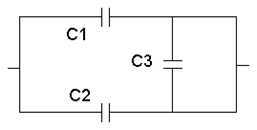# Electrostatic Potential And Capacitance

15 Questions | Attempts: 2701
ShareSettingsTime: 30 Minute

• 1.
A parallel plate capacitor has a capacitance of 50 pf in air and 105 pf when immersed in oil. The dielectric constant of the oil is:
• A.

50/105

• B.

1

• C.

2.1

• D.
• 2.
Three capacitors of capacitance 4µ F, 6µF and 12µF are connected first in series and then in parallel. What is the ratio of equivalent capacitance in the two cases?
• A.

2 : 3

• B.

1 : 11

• C.

11 : 1

• D.

1 : 3

• 3.
A work of 100 joule is performed in carrying a charge of -5 coulomb from infinity to a particular point in an electrostatic field. The potential of this point is:
• A.

100 V

• B.

5 V

• C.

-20 V

• D.

20 V

• 4.
When air is replaced by a dielectric medium of constant K, the maximum force of attraction between two charges separated by a distance:
• A.

Becomes times

• B.

Increases K times

• C.

Remains unchanged

• D.

Decreases K times

• 5.
Potential energy of two equal negative point charges 2µC each held 1m apart in air is:
• A.

2 J

• B.

2 eV

• C.

4 J

• D.

0.036 J

• 6.
The electric potential at the surface of an atomic nucleus (Z = 50) of radius 9.0 x 10-15 m is:
• A.

80 volt

• B.

8 x  volt

• C.

9 volt

• D.

9 x  volt

• 7.
Three different capacitors are connected in series. Then:
• A.

They will have equal charges

• B.

They will have same potential

• C.

Both 1 & 2

• D.

None of these

• 8.
A 2µF capacitor is charged to 100 volt and then its plates are connected by a conducting wire. The heat produced is:
• A.

0.001 J

• B.

0.01 J

• C.

0.1 J

• D.

1 J

• 9.
A hollow metal sphere of radius 10 cm is charged such that the potential on its surface is 80 volt. The potential at the centre of the sphere is:
• A.

8 volt

• B.

800 volt

• C.

80 volt

• D.

Zero

• 10.
A parallel plate capacitor is made by stocking n equally spaced plates connected alternately. If the capacitance between any two plates is x, then the total capacitance is:
• A.

Nx

• B.

N/x

• C.

X

• D.

(n – 1) x

• 11.
The equivalent capacity of the combination C1 = C2 = C3 shown in figure is:
• A.

2 C

• B.

C

• C.

C / 2

• D.

None of these

• 12.
Three capacitors each of capacity 4 µF are to be connected in such a way that the effective capacitance is 6 µF. This can be done by:
• A.

Connecting all of them in series

• B.

Connecting all of them in parallel

• C.

Connecting two in series and one in parallel

• D.

Connecting two in parallel and one in series

• 13.
The magnitude of electric field E in the annular region of a charged cylindrical capacitor.
• A.

Is the same throughout

• B.

Is higher near the outer cylinder than near the inner cylinder

• C.

Varies as 1/r, where r is the distance from the axis

• D.

Varies as 1/ , where r is the distance from the axis.

• 14.
A 800 µF capacitor is charged at the steady rate of 50 µC/sec. How long will it take to raise its potential to 10 volt?
• A.

160 s

• B.

50 s

• C.

10 s

• D.

500 s

• 15.
If there are n capacitors in parallel connected to V volt source, then energy stored is equal to:
• A.

CV

• B.

C

• C.
• D.

nC

## Related TopicsBack to top
×

Wait!
Here's an interesting quiz for you.